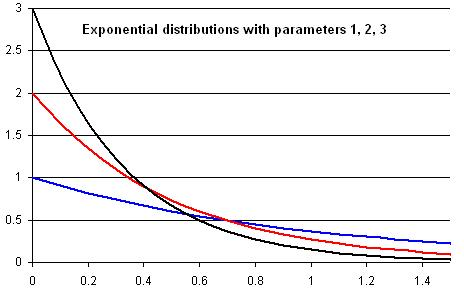#### You may also like### PDF

Given a probability density function find the mean, median and mode of the distribution.### Scale Invariance

By exploring the concept of scale invariance, find the probability that a random piece of real data begins with a 1.### PCDF

When can a pdf and a cdf coincide?

# Into the Exponential Distribution

### Why do this problem?

This problem is a sequence of activities based around the exponential distributions. The aim is to draw the learner into an understanding of the properties of pdfs without requiring too many complicated calculations: it uses and will reinforce ideas about functions, integration and areas. It will also suit self-motivated independent learners.

### Possible approach

The questions start off very simply and would lead to good group discussion. You may wish to use only parts of the question and it would be of value when students have encountered pdfs but have not necessarily had much experience with their manipulation. Some of the questions naturally ask 'why' a result is true. Learners should be encouraged to think about these, but not get too concerned if they can't come up with a $100\%$ watertight answer. The key is to understand that the results intuitively must be true and get into calculating and estimating probabilities from a pdf.

### Key questions

What do you know about the areas under a pdf?
Are there any turning points? What does this tell you?

### Possible extension

Can students analyse the different areas formed by these three exponential curves?### Possible support

Encourage learners to rely on their intuitive understanding of integration in terms of area. Alternatively, focus on the last two parts of the question as a discussion.